# Loop inside or outside a script?

Dear knimers,

Imagine you have to import a bunch of files (say 100) into your knime workflow. The file format contains 10000 floating point numbers (doubles). Unfortunately, the files are in some weird proprietary file format. Fortunately, for almost every proprietary file format someone made a Python library that understands the file format. So the obvious solution is to write a small Python script that reads the file and returns a Pandas dataframe that Knime understands. But we are not dealing with a single file, we are dealing with whole bunch of files. Question is, should we loop over those files using a Knime loop or should we put the loop in the Python script?

Let’s simulate this situation by generating random numbers inside a Python script. To keep the workflow scalable, the information for every new iteration (file) should be put in a new table row, not in a new column. And, instead of putting the values of one iteration in 10000 columns, we put all 10000 values in a single list cell.

The Python script without the loop then looks like:

``````import numpy as np

n = 10000

output_table = input_table.copy()
z = np.random.randn(1,n).tolist()
output_table = output_table.assign(Out=z)
``````

The corresponding Knime workflow looks like: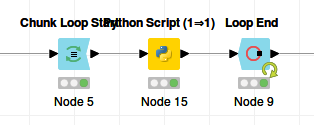We are simulating loading one file per iteration, so the chunk size is 1.

If we include the loop in the Python script, the script becomes:

``````import numpy as np

n = 10000

output_table = input_table.copy()
z = np.zeros((len(input_table),n)).tolist()
output_table = output_table.assign(Out=z)

for i, row in output_table.iterrows():
row['Out'] = np.random.randn(1,n).tolist()
output_table.loc[i] = row
``````

In this case the Knime workflow is only the Python script: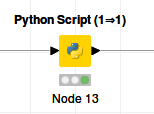In both cases the output looks like: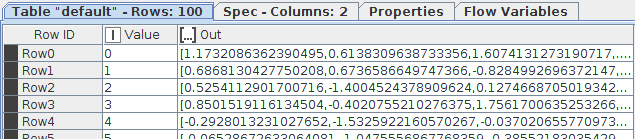The first method takes 173 seconds.
The second methods takes 4 seconds.

How about 100 x 100000 values?
In this case the first method takes option takes 198 seconds.
The second methods takes 26 seconds.

Conclusion: if workflow execution speed matters, it is absolutely worthwhile to write the Python script in such a way that it includes the loop. It is obvious that launching and exiting the Python environment, and serializing and deserializing the data takes so much time that this should be limited as much as possible.

It should be noted that because Python/numpy/pandas does everything in memory, one may run out of memory for large datasets. In this case it would be wise to have both a loop in the Python code and a Knime Chunk Loop with a reasonably large but not too large chunk size.

The workflow: KNIME_project2.knwf (27.2 KB)

4 Likes

It would be interesting to modify your example to store floats in your pandas.DataFrame instead of storing a Python list in a single cell as you have it now; because your current approach stores a Python object (the list) rather than leveraging the speed of native dtypes (e.g. np.float64) in pandas, you are giving up most of the performance that pandas offers. Note this will benefit your Python code (independent of KNIME) as well as your work between Python and KNIME.

Also when working with pandas DataFrames, iterating over rows (iterrows()) is drastically more expensive than iterating over columns or individual values within a column. There are situations where it is necessary, but the use of iterrows() should be regarded as a “choice of last resort” when working with pandas DataFrames. Your example can easily be rewritten to avoid the use of iterrows().

I hope the above suggestions (which have nothing to do with KNIME but everything to do with helping everyone write good performing code with pandas) prove to be helpful pointers especially given you are clearly interested in performance matters in this post.

There will be situations where, as in your particular example, it is advantageous to put a loop inside the Python script, and there will be different situations where it is advantageous to keep the loop outside of the Python code and use the Looping nodes instead. I would suggest that the correct conclusion / recommendation is to test both scenarios when preparing KNIME workflows for production use – what works best for a given workflow and its data is difficult to predict without testing and the impact to performance could be larger or smaller than one might expect.

4 Likes

@potts, thank you for your comment. I wasn’t aware that growing a pandas dataframe sideways (adding columns) is faster than growing it downwards (adding rows). However, even though I chose the less optimal method for constructing a pandas dataframe, and even though I insert lists into the dataframe cells instead of np.float64s, the Python code in the examples above executes in less than a second. The rate-limiting step is clearly the interaction between Knime and Python.

I also tried constructing the list cells in Knime instead of in Python, by concatenating the datasets top-down and adding a group index. This way I only need to use normal floats in the Pandas dataframe. Subsequently I convert these into lists by using the GroupBy node:This takes 14 seconds.

One can also do this by creating a 10000 column wide dataframe in Python and then converting it to lists within Knime by using a Create Collection Column node: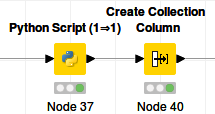This takes 5.5 seconds, slower but but not that much slower than the 4 seconds in my first post. That’s nice, because inserting lists into Python dataframe cells is a bit ugly I admit.

However, taking the loop out of the script slows the whole thing down again: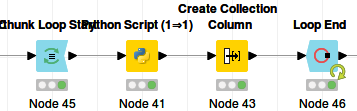225 seconds.

2 Likes

Instead of 100 rows, let’s create 1024 rows of 10000 numbers. And let’s loop both in the Python script and with the Knime Chunk loop construct. If we vary the number of iterations assigned to Python and to Knime, perhaps we can find an optimal division of labour between the two.

Chunk size 1024: 27 seconds (1 iteration in Knime, 1024 iterations in Python)
Chunk size 512: 29 seconds (2 iterations in Knime, 512 iterations in Python)
Chunk size 256: 32 seconds (4 iterations in Knime, 256 iterations in Python)
Chunk size 128: 39 seconds
Chunk size 64: 53 seconds
Chunk size 32: 80 seconds
Chunk size 16: 134 seconds
Chunk size 8: 247 seconds
Chunk size 4: 466 seconds

Here the optimal division of labour is letting Python do all the workI think this is the case as long as Python has memory available. I would love to see an example where the opposite is true…

This topic was automatically closed 182 days after the last reply. New replies are no longer allowed.